python系列 文件操作的代碼

更新時間：2019年10月06日 21:04:55   作者：Fengqiao_x我要評論

import numpy as np
import os,sys

#獲取當前文件夾，并根據文件名
def path(fileName):
p=sys.path+'\\'+fileName
return p

#讀文件
f=open(path(fileName))
f.close()
return str

#寫文件
def writeFile(fileName,str):
f=open(path(fileName),'w')
f.write(str)
f.close()

def str1():
str=','.join('我在中國大地上驕傲地生長著！')
return str

def str2():
return str(np.random.randint(-49,50,[3,3,3]))

#實驗1
def test_1():
fileName='中國大地.txt'
writeFile(fileName,str1())
print(list)

#實驗2
def test_2():
writeFile('str1',str1())
writeFile('str2',str2())
print(str_1)
print(str_2)

test_2()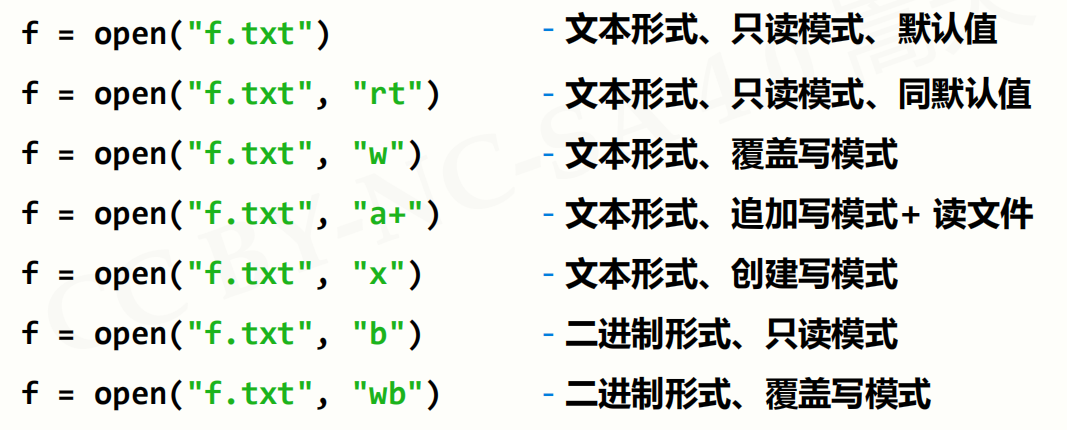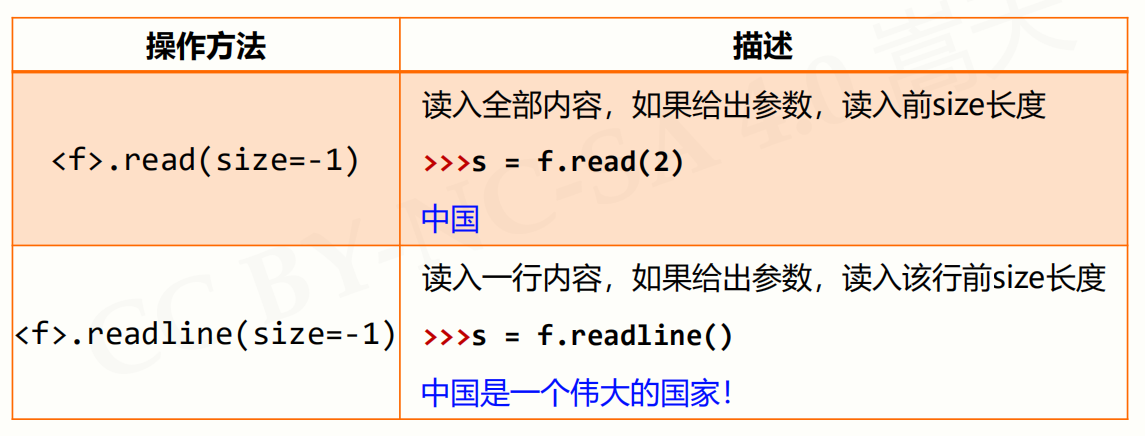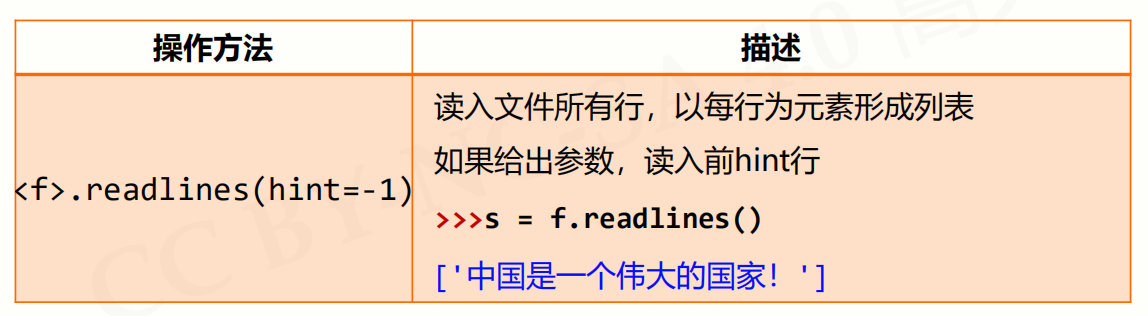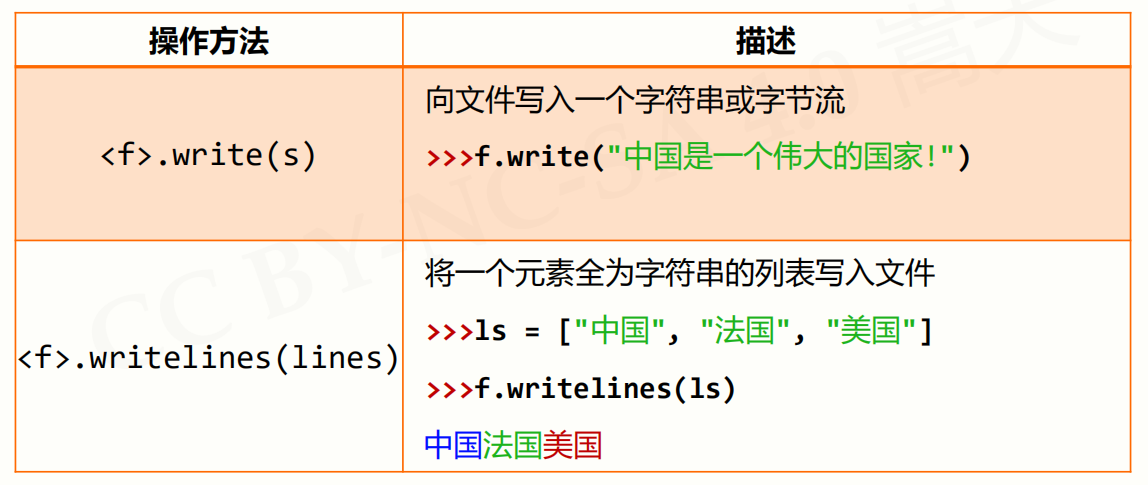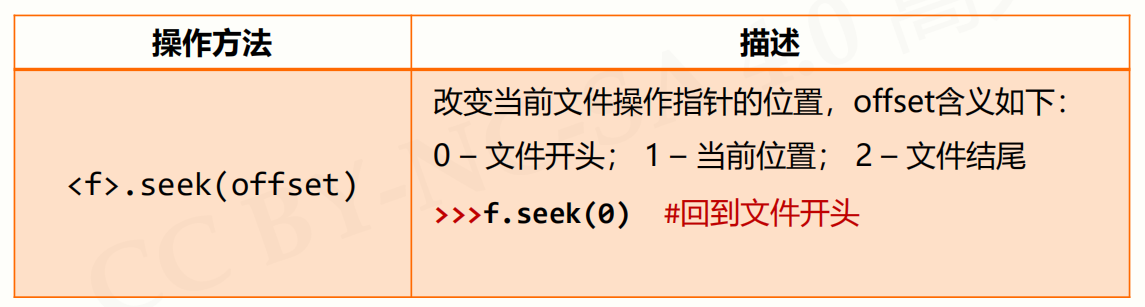randint(low[,high,shape]) 根據shape創建隨機整數或整數數組，范圍是[low, high)

numpy.random.randint的詳細用法

numpy.random.randint(low, high=None, size=None, dtype='l')

low: int 生成的數值最低要大于等于low。
（hign = None時，生成的數值要在[0, low)區間內）
high: int (可選) 如果使用這個值，則生成的數值在[low, high)區間。
size: int or tuple of ints(可選) 輸出隨機數的尺寸，比如size=(m * n* k)則輸出同規模即m * n* k個隨機數。默認是None的，僅僅返回滿足要求的單一隨機數。
dtype: dtype(可選)： 想要輸出的格式。如int64、int等等

>>> np.random.randint(2, size=10)
array([1, 0, 0, 0, 1, 1, 0, 0, 1, 0])
>>> np.random.randint(1, size=10)
array([0, 0, 0, 0, 0, 0, 0, 0, 0, 0])
>>> np.random.randint(5, size=(2, 4))
array([[4, 0, 2, 1],
[3, 2, 2, 0]])
>>>np.random.randint(2, high=10, size=(2,3))
array([[6, 8, 7],
[2, 5, 2]])